# Introduction

The `robvis` package provides functions to convert a risk-of-bias assessment summary table into a summary plot or a traffic-light plot, formatted based on the specific risk-of-bias assessment tool used.

`robvis` currently contains templates for the following tools:

• ROB2
• ROBINS-I
• QUADAS-2
• ROB1

Users can find the exact assessment tool name expected by the `tool` argument of the `rob_summary()` and `rob_traffic_light()` functions by running:

``````rob_tools()
#>  "ROB2"
#>  "ROBINS-I"
#>  "QUADAS-2"
#>  "ROB1"``````

## Loading your data

`robvis` expects certain facts about the data you provide it.

1. The first column contains the study identifier
2. The second-to-last column will contain the overall risk-of-bias judgments
3. The last column will contain the weights, which can all be set to 1 if the relevant weights are not available.
4. The first row of the data does not contain column headings. This can be achieved using the `header = TRUE` option (which indicates that the first line contains column headings) when reading in your summary table:
``data <- read.csv("path/to/summary_table.csv", header = TRUE)``

All other columns are expected to contain the results of the risk-of bias assessments for a specific domain. To elaborate, consider as an example the ROB2.0 tool which has 5 domains. The resulting data set that `robvis` would expect for this tool would have 8 columns:

• Column 1: Study identifier
• Column 2-6: One RoB2 domain per column
• Column 7: Overall risk-of-bias judgments
• Column 8: Weights

The only exception to this is the `"ROB1"` template, which is discussed below.

## Example data sets

To help users explore `robvis`, we have included an example data set for each tool template that exists in the package. For example, the `data_rob2` data set, which contains example risk-of-bias assessments performed using the RoB2.0 tool for randomized controlled trials, is presented below:

Study D1 D2 D3 D4 D5 Overall Weight
Study 1 Low Low Low Low Low Low 33.3333333
Study 2 Some concerns Low Low Low Low Low 33.3333333
Study 3 Some concerns Low Some concerns Low Low Some concerns 0.1428571
Study 4 Low Low High Low Some concerns High 9.0909091
Study 5 High High Low Low Some concerns Low 12.5000000
Study 6 Low High Some concerns Low Low Some concerns 25.0000000
Study 7 Low Some concerns Some concerns High Low Some concerns 200.0000000
Study 8 Low Some concerns Low Low Low Low 11.1111111
Study 9 Low Low High Low Low High 1.1111111

# Summary plots (`rob_summary()`)

This function returns a `ggplot` object displaying a weighted bar-chart of the distribution of risk-of-bias judgments across the domains of the specified tool.

## Examples:

### RoB2.0 tool for randomized controlled trials

``rob_summary(data_rob2, tool = "ROB2")``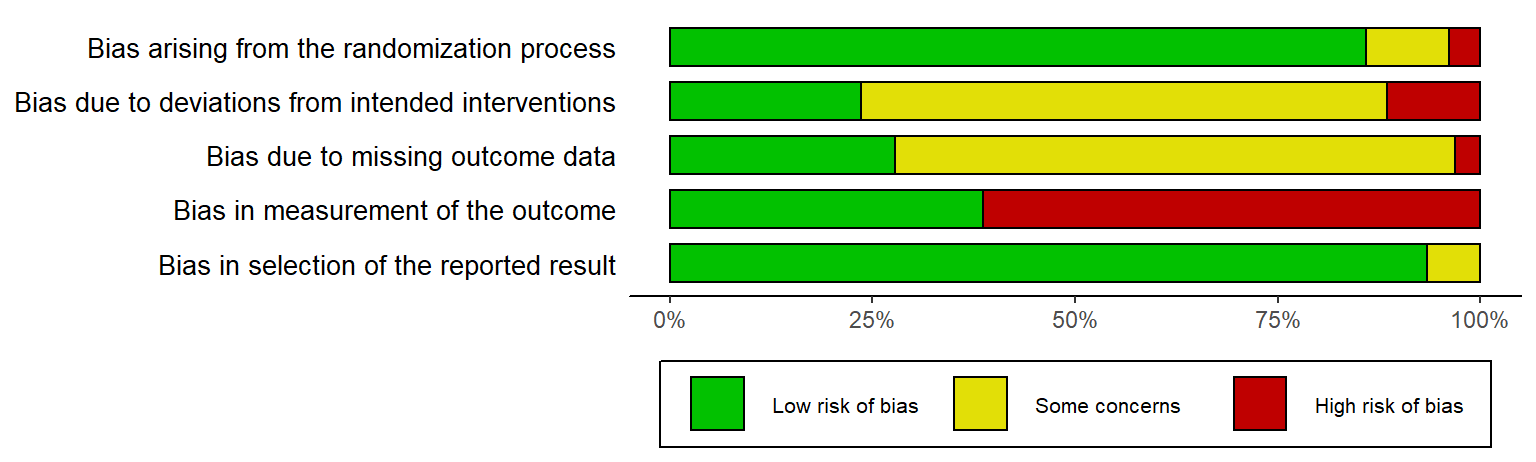### ROBINS-I tool for non-randomized studies of interventions

``rob_summary(data_robins, tool = "ROBINS-I")``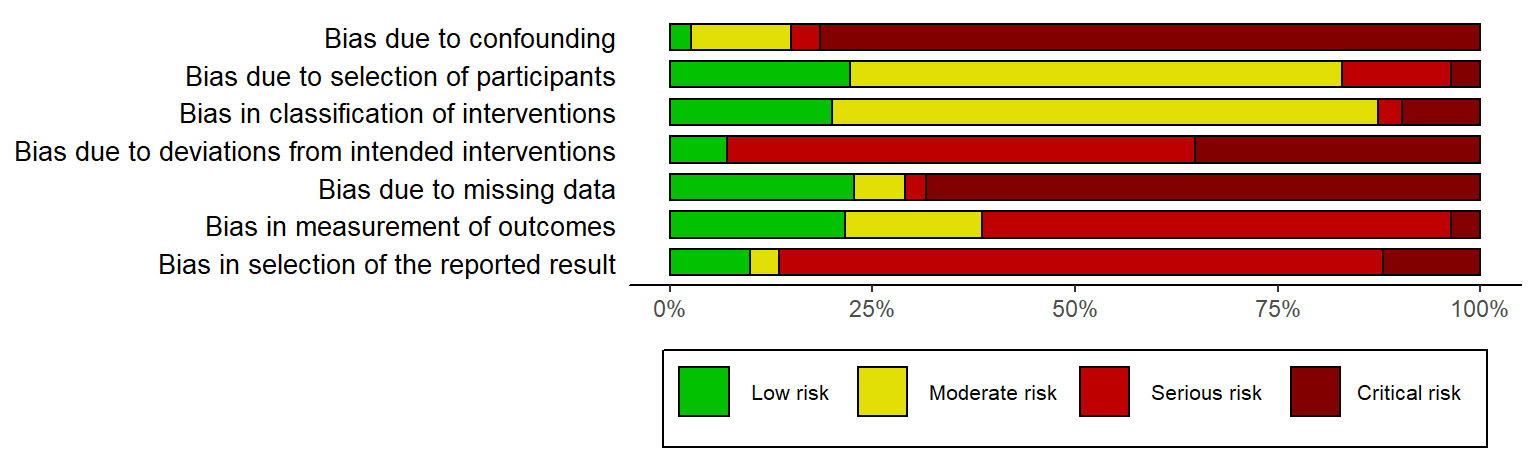### QUADAS-2 tool for diagnostic test accuracy studies

``rob_summary(data_quadas, tool = "QUADAS-2")``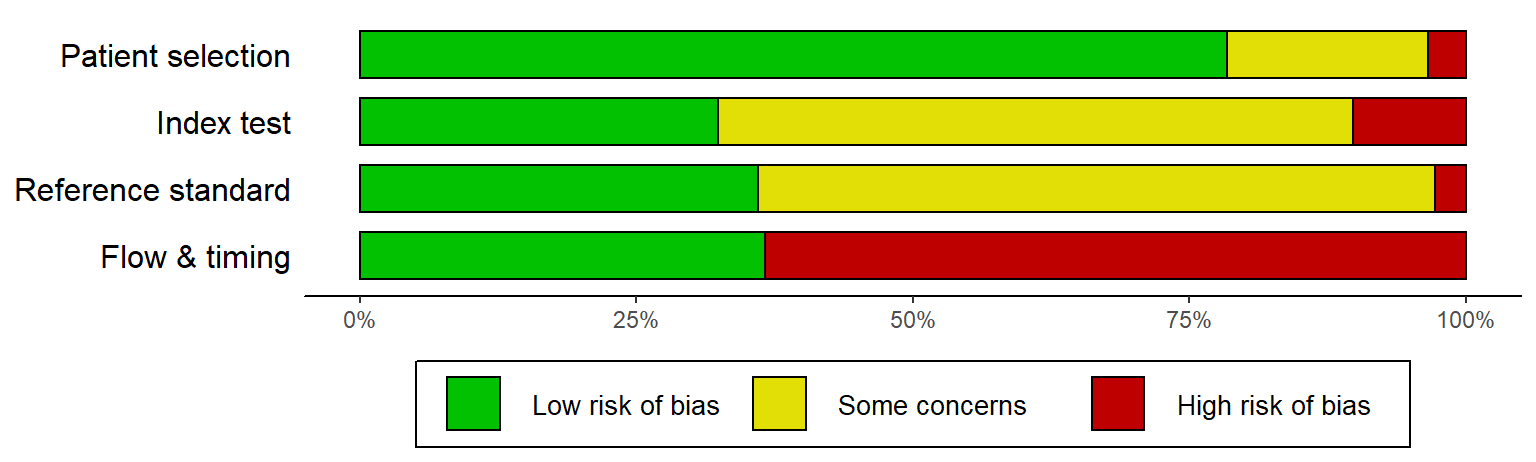## `rob_summary()` options

### Overall risk-of-bias judgments (`overall`)

By default, a bar representing the overall risk-of-bias judgments is not included in the plot. If you would like to include this, set `overall = TRUE`. For example:

``rob_summary(data_rob2, tool = "ROB2", overall = TRUE)``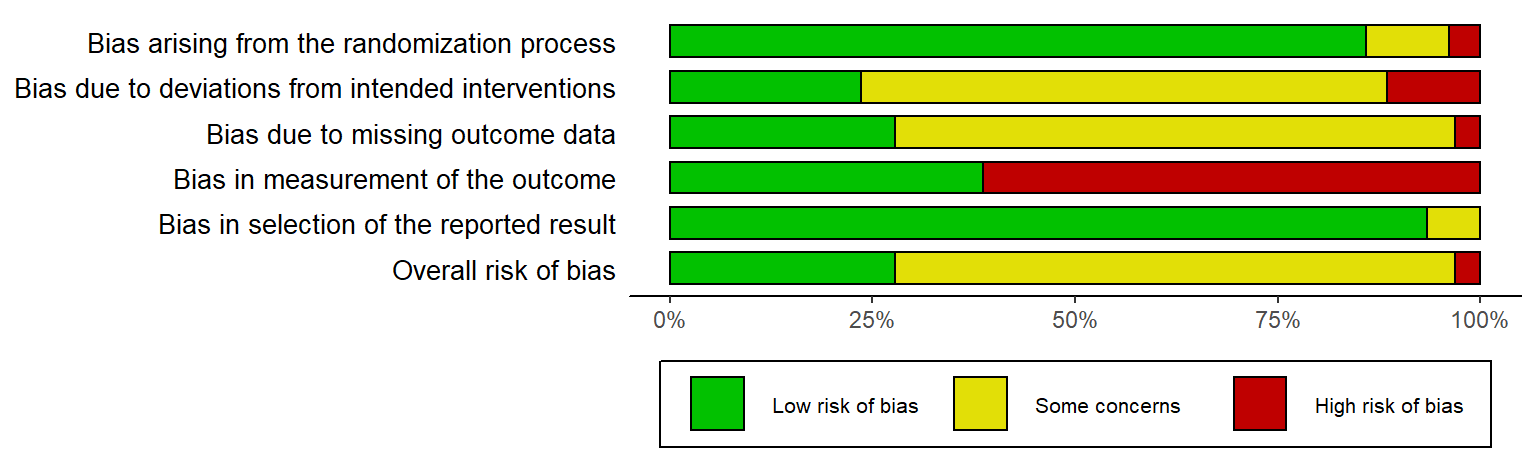### Weighted or un-weighted bar plots (`weighted`)

By default, the barplot is weighted by some measure of study precision (see Example data sets). You can turn off this option by setting `weighted = FALSE`. For example, compare this plot with that produced by the based `rob_summary()` function using the `data_rob2` data set.

``rob_summary(data_rob2, tool = "ROB2", weighted = FALSE)``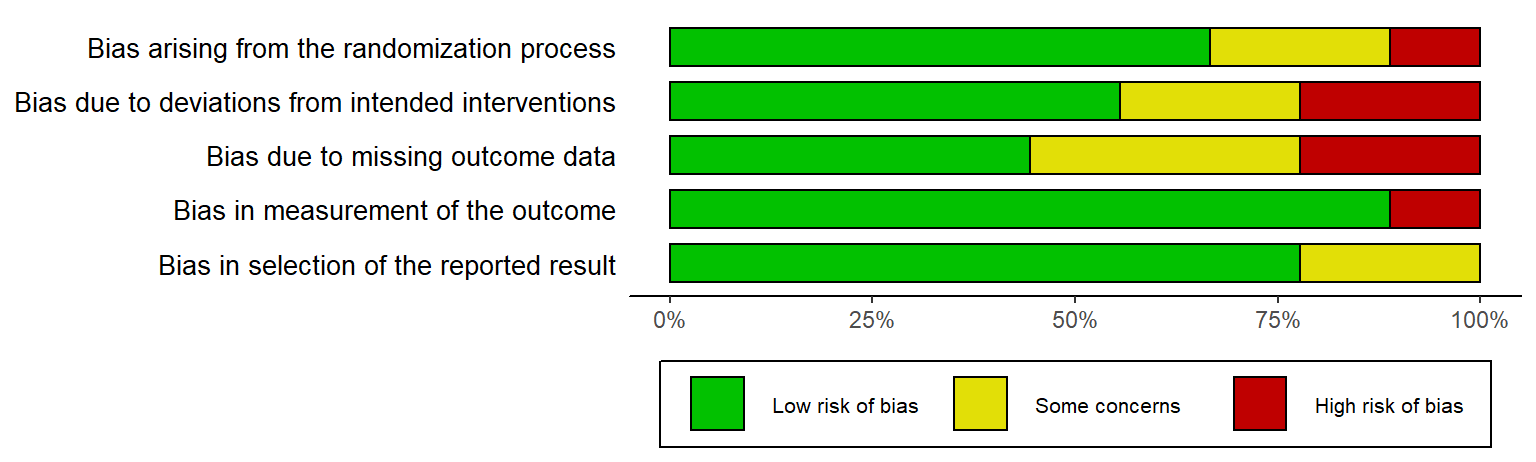### Colour scheme (`colour`)

NB: Please note the non-US English spelling of colour

The `colour` argument of both plotting functions allows users to select from two predefined colour schemes, “cochrane” (default) or “colourblind”, or to define their own palette by providing a vector of hex codes.

For example, to use the predefined “colourblind” palette:

``rob_summary(data = data_rob2, tool = "ROB2", colour = "colourblind")``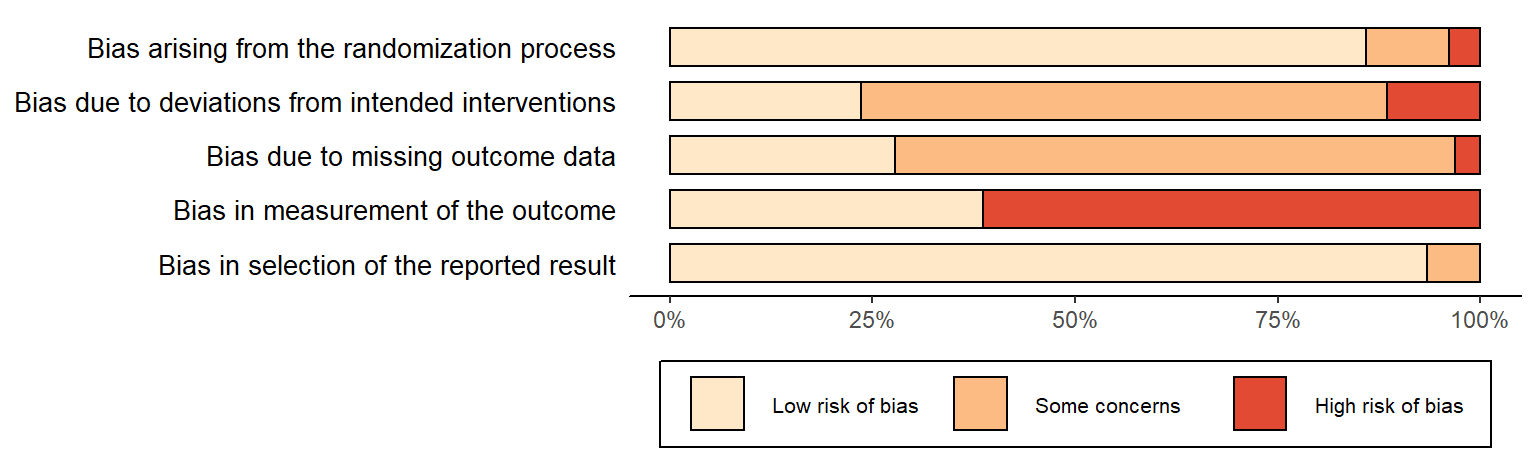And to define your own colour scheme:

``rob_summary(data = data_rob2, tool = "ROB2", colour = c("#f442c8","#bef441","#000000"))``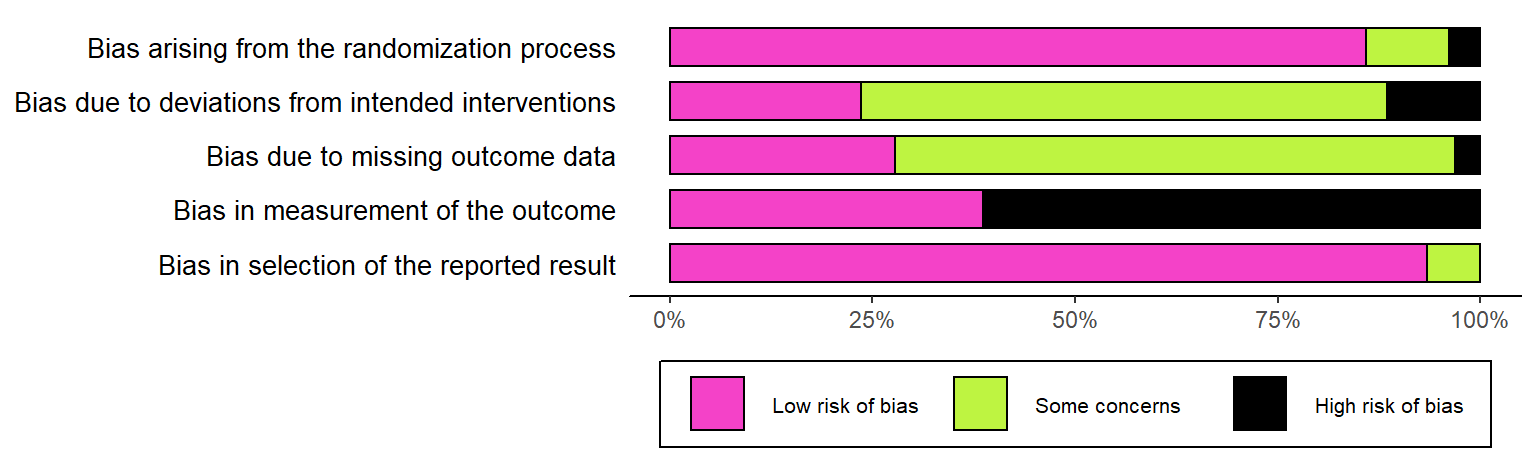When defining your own colour scheme, you must ensure that the number of discrete judgments (e.g. “Low”/“Moderate”/“High”/“Critical”) and the number of colours specified are the same. Additionally, colours must be specified in order of ascending risk-of-bias (e.g. “Low” -> “Critical”), with the first hex corresponding to “Low” risk of bias.

# Traffic light plots (`rob_traffic_light()`)

This function returns a `ggplot` object displaying the risk-of-bias judgment in each domain for each study, as well as the overall risk-of-bias judgement for that study.

## Examples:

### RoB2.0 tool for randomized controlled trials

``rob_traffic_light(data_rob2, tool = "ROB2")``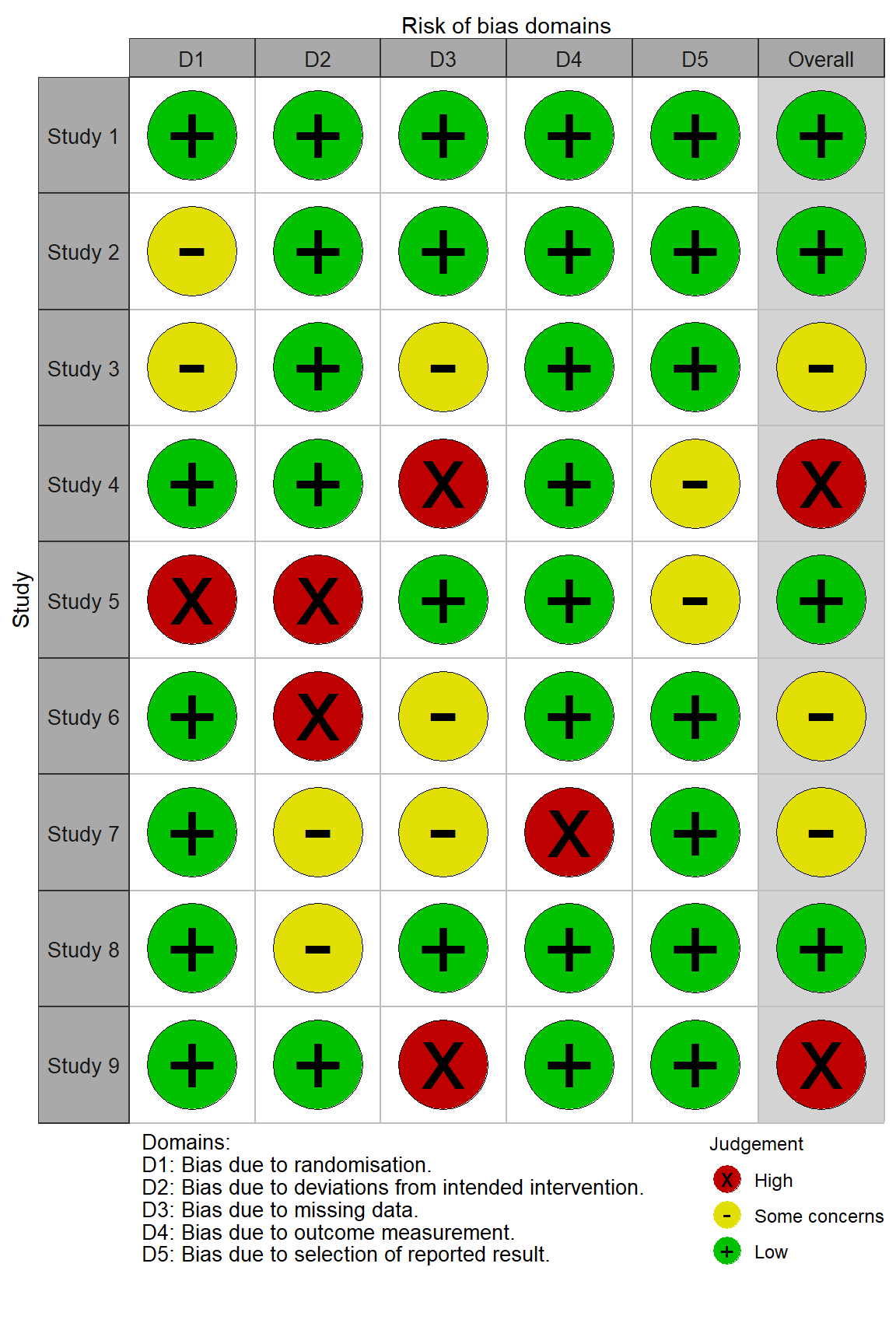### ROBINS-I tool for non-randomized studies of interventions

``rob_traffic_light(data_robins, tool = "ROBINS-I")``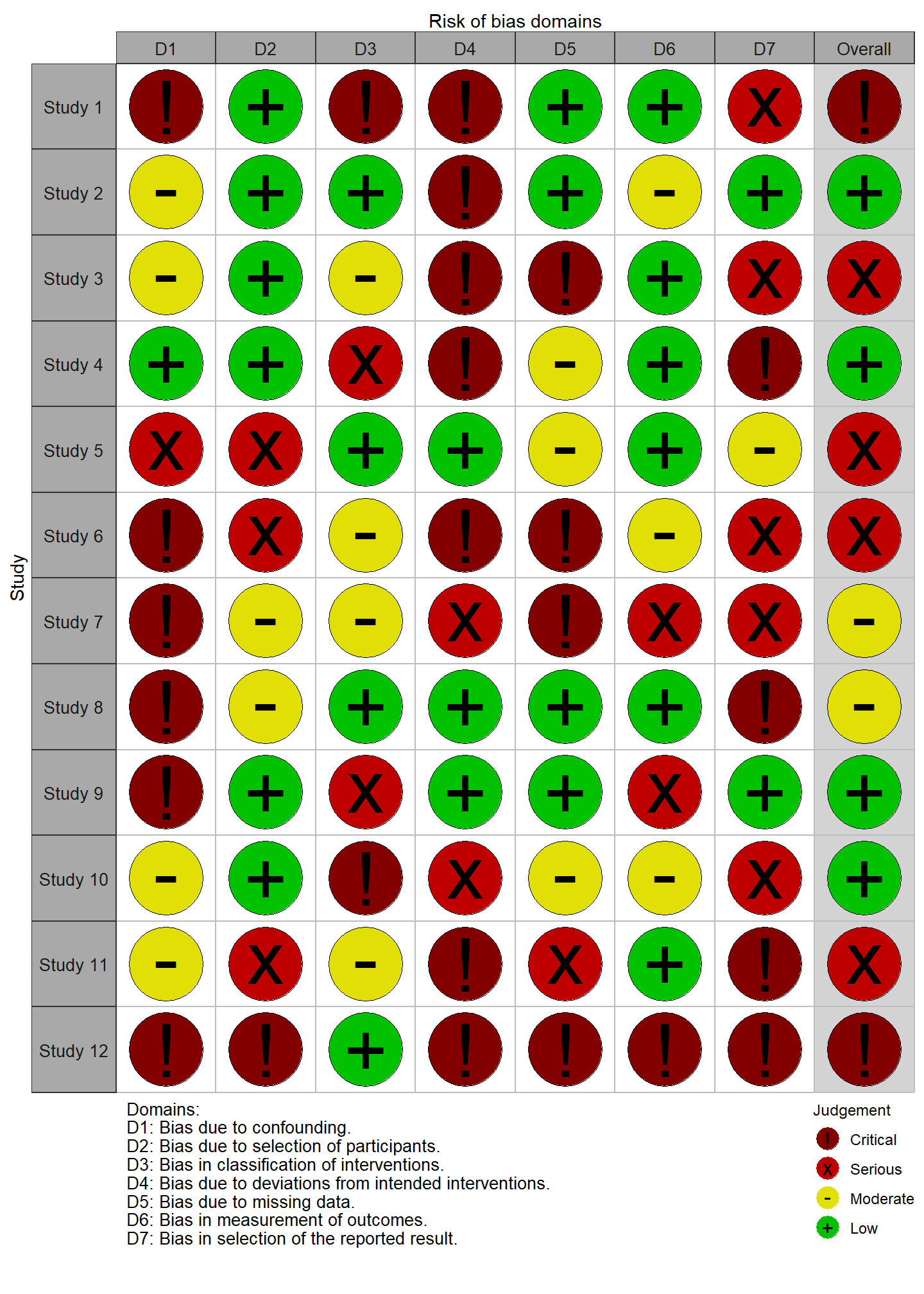### QUADAS-2 tool for diagnostic test accuracy studies

``rob_traffic_light(data_quadas, tool = "QUADAS-2")``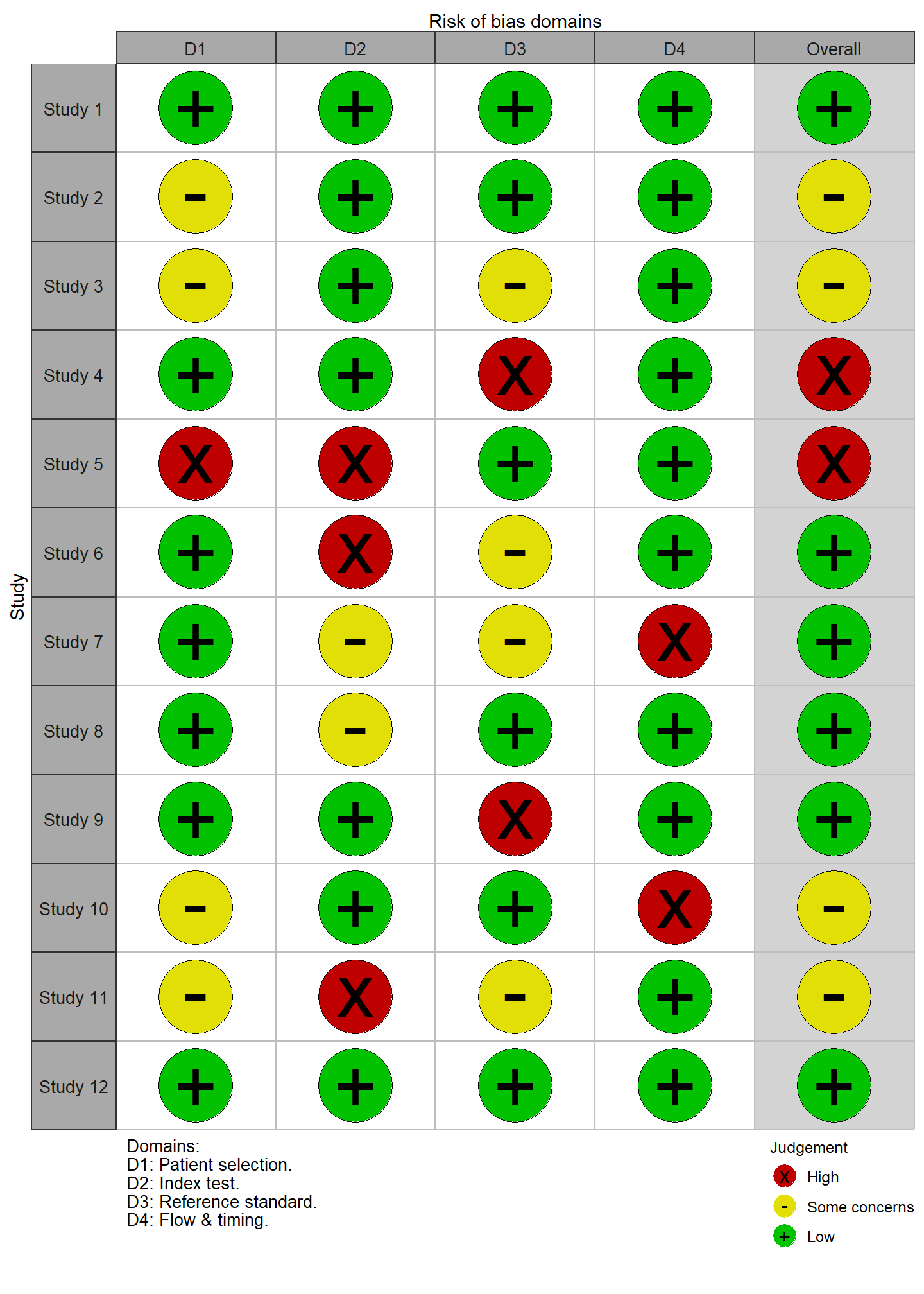## `rob_traffic_light()` options

### Point size (`psize`)

By default, the size of each point is set to 20. However, if you have a large number of studies, it is useful to be able to reduce the point size so that the resulting graphic is not too large.

``````# Generate larger dataset
data <- rbind(data_rob2, data_rob2)
data\$Study <- paste("Study",seq(1,18))

# Plot with reduced point size
rob_traffic_light(data, tool = "ROB2", psize = 10)``````